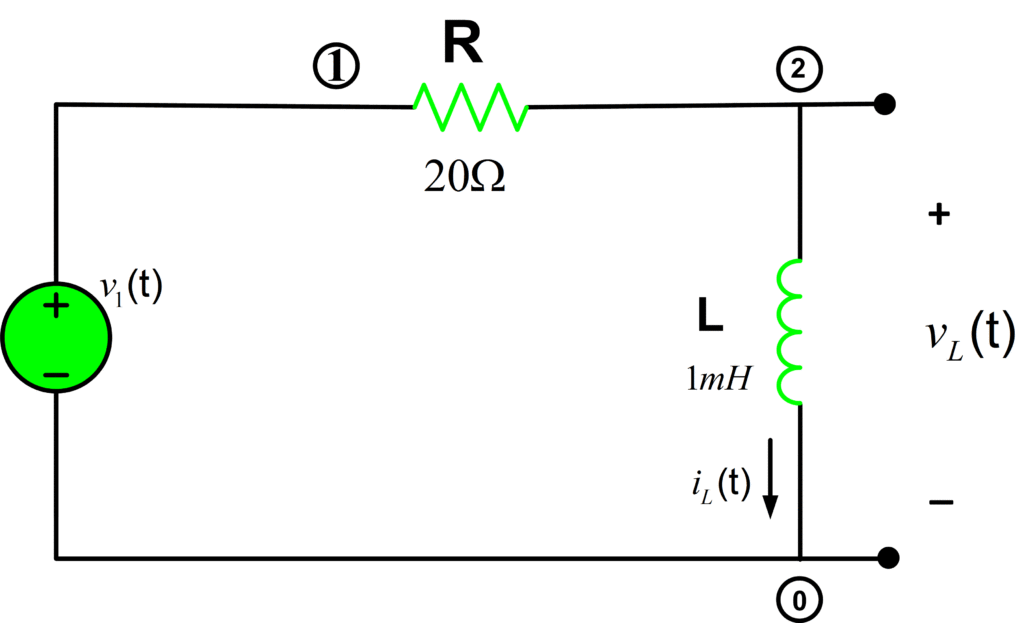Home / Circuits with Matlab / Series RL Circuit Analysis using Matlab

# Series RL Circuit Analysis using Matlab

Want create site? Find Free WordPress Themes and plugins.

Determine the voltage across the inductor in series RL circuit :Let us compute the voltage across the capacitor for t≥0 using the following expression:

${{v}_{L}}(t)={{V}_{s}}{{e}^{-t/\tau }}u(t)$

Whereas the source voltage is 1V and time constant τ=L/R.

It’s time to write some code in Matlab to calculate the inductor voltage:

%RL Circuit Analysis
clear all;close all;clc
%%Circuit Parameters
R= 20; % Resistance (20 Ohm)
L= 1e-3; % Inductance (1mH)
tau= L/R; % RL Circuit Time Constant
Vs= 1; % Source Voltage (1V)
Time= 0:tau/100:3*tau; % Sampling Time
V_L= Vs.*exp(-Time./tau).*heaviside(Time);
plot(Time,V_L)
xlabel('Time (s)')
ylabel('Amplitude (V)')
title('V_L')
%=============================================


Results:Did you find apk for android? You can find new Free Android Games and apps.